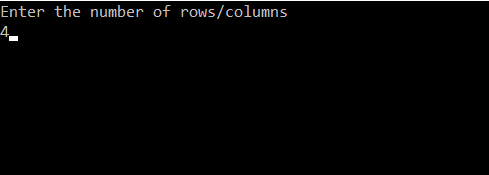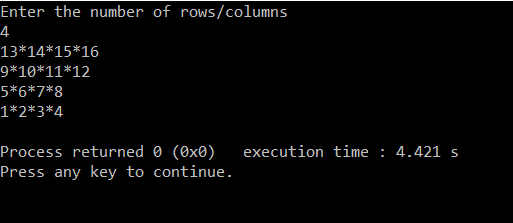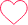# Print Number Star Square Pattern Type2

13*14*15*16

9*10*11*12

5*6*7*8

1*2*3*4

## PREREQUISITE:

Basic knowledge of C language and use of loops.

## ALGORITHM:

1. Take the number of rows/columns as input from the user and store it in any variable.(‘l‘ in this case).
2. Run a loop ‘l’ number of times to iterate through each of the rows. From i=0 to i<l. The loop should be structured as for( i=0 ; i<l : i++).
3. Inside this loop run another nested loop to iterate through the columns. From j=0 to j<l. The loop should be structured as for(j=0 ; j<l ; j++).
4. And increment count to get the max number required.
5. Run a loop ‘l’ number of times to iterate through each of the rows. From i=0 to i<l. The loop should be structured as for( i=0 ; i<l : i++).
6. Inside this loop run another nested loop to iterate through the columns. From j=0 to j<l. The loop should be structured as for(j=0 ; j<l ; j++).
7. increment count and run an if condition if(j==l-1).
8. if true then print count else print count and a star after it.
9. outside the nested loop reinitialize count=count-2*l then print a newline

## CODE IN C:

```#include<stdio.h>
int main()
{
int i,j,l,count=0; //declaring integers i,j for loops and l for number of rows
printf("Enter the number of rows/columns\n"); //Asking user for input
scanf("%d",&l); //Taking the input for number of rows
for(int i=0;i<l;i++) //Outer loop for number of rows
{
for(j=0;j<l-1;j++)
{
count++;
}
}
for(i=0;i<l;i++)
{
for(j=0;j<l;j++)
{
count++;
if(j==l-1)
{
printf("%d",count);
}
else
{
printf("%d*",count);
}

}
count=count-2*l;
printf("\n");
}

}```

## TAKING INPUT:DISPLAYING OUTPUT:### 3 comments on “Print Number Star Square Pattern Type2”

•Bhupendra

#include
using namespace std;

int main()
{
int n;
cin>>n;
int count=n*n;
for (int i=1; i<=n; i++){
count=count-4*i+1;
for (int j=1; j<=n; j++){
cout<<count++<<"*";
}
count=n*n;
cout<<endl;
}

return 0;
}0
•Bhupendra

if 4 replace by n, then it will work for all numbers.0
•Prasoon

#include
using namespace std;

int main() {
int rows,count=13;
cout<>rows;
for(int i=0; i<rows; i++) {
for(int j=0; j<rows; j++) {
cout<<count++<<"*";
}
count = count-8;
cout<<"\n";
}
return 0;
}0